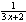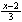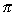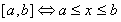Next: Limits Up: Topics to Study Previous: Topics to Study

## Elementary Functions

Properties of Functions

A function ƒ is defined as a set of all ordered pairs (x, y), such that for each element x, there corresponds exactly one element y.

The domain of ƒ is the set x.

The range of ƒ is the set y.

Combinations of Functions

If ƒ(x) = 3x + 1 and g(x) = x2 - 1

a) the sum ƒ(x) + g(x) = (3x + 1) + (x2 - 1) = x2 + 3x

b) the difference ƒ(x) - g(x) = (3x + 1) - (x2 - 1) = -x2 + 3x + 2

c) the product ƒ(x)g(x) = (3x + 1)(x2 - 1) = 3x3 + x2 - 3x - 1

d) the quotient ƒ(x)/g(x) = (3x + 1)/(x2 - 1)

e) the composite (ƒ ° g)(x) = ƒ(g(x)) = 3(x2 - 1) + 1 = 3x2 - 2

Inverse Functions

Functions ƒ and g are inverses of each other if

ƒ(g(x)) = x for each x in the domain of g

g(ƒ(x)) = x for each x in the domain of ƒ

The inverse of the function ƒ is denoted ƒ-1.

To find ƒ-1, switch x and y in the original equation and solve the equation for y in terms of x.

 Exercise: If ƒ(x) = 3x + 2, then ƒ-1(x) = (A)(B)- 2 (C) 3x - 2 (D)x + 3 (E)The answer is E. x = 3y + 2 3y = x - 2 y =Even and Odd Functions

The function y = ƒ(x) is even if ƒ(-x) = ƒ(x).

Even functions are symmetric about the y-axis (e.g. y = x2)

The function y = ƒ(x) is odd if ƒ(-x) = -ƒ(x).

Odd functions are symmetric about the origin (e.g. y = x3)

 Exercise: If the graph of y = 3x + 1 is reflected about the y-axis, then an equation of the reflection is y = (A) 3x - 1 (B) log3 (x - 1) (C) log3 (x + 1) (D) 3-x + 1 (E) 1 - 3x The answer is D. The reflection of y = ƒ(x) in the y-axis is y = ƒ(-x)

Periodic Functions

You should be familiar with the definitions and graphs of these trigonometric functions:

sine, cosine, tangent, cotangent, secant, and cosecant

 Exercise: If ƒ(x) = sin(tan-1 x), what is the range of ƒ? (A) (-/2,/2) (B) [-/2,/2] (C) (0, 1] (D) (-1, 1) (E) [-1, 1] The answer is D. The range of sin x is (E), but the points at which sin x = 1 (/2 + k), tan-1 x is undefined. Therefore, the endpoints are not included.

Note: The range is expressed using interval notation:Zeros of a Function

These occur where the function ƒ(x) crosses the x-axis. These points are also called the

roots of a function.

 Exercise: The zeros of ƒ(x) = x3 - 2x2 + x is (A) 0, -1 (B) 0, 1 (C) -1 (D) 1 (E) -1, 1 The answer is B. ƒ(x) = x(x2 - 2x + 1) = x(x -1)2

Properties of Graphs

You should review the following topics:

a) Intercepts

b) Symmetry

c) Asymptotes

d) Relationships between the graph of

 y = ƒ(x) and y = kƒ(x) y = ƒ(kx) y - k = ƒ(x - h) y = |ƒ(x)| y = ƒ(|x|)Next: Limits Up: Topics to Study Previous: Topics to Study